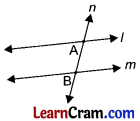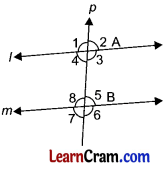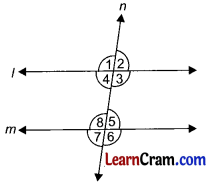# DAV Class 6 Maths Chapter 11 Worksheet 1 Solutions

The DAV Maths Book Class 6 Solutions and DAV Class 6 Maths Chapter 11 Worksheet 1 Solutions of Transversal and Pairs of Lines offer comprehensive answers to textbook questions.

## DAV Class 6 Maths Ch 11 WS 1 Solutions

Question 1.
Tick (✓) the figures in which the line ‘t’ is a transveral to the other given lines.(c) ✓
(f) ✓

### DAV Class 6 Maths Chapter 11 Worksheet 1 Notes

A line which intersects any two or more lines is called ‘transversal line’.
In the given figure, line n intersects the lines l and m respectively at A and B.n is the transversal line.

Angles made by a Transversal with two or more Lines• When a transversal line intersects any two lines at different points, it makes 8 angles with the lines. Here transversal line p intersects the two lines Z and m at A and B respectively.
• It makes the following angles with l and m ∠1, ∠2, ∠3, ∠4, ∠5, ∠6, ∠7 and ∠8.
• Interior angles: ∠3, ∠4, ∠5 and ∠8 are interior angles.
• Exterior angles: ∠1, ∠2, ∠6 and ∠7 are exterior angles.
• Corresponding angles: ∠2 and ∠5, ∠3 and ∠6, ∠1 and ∠8, ∠4 and ∠7 are corresponding angles.
• Alternate interior angles: ∠3 and ∠8, ∠4 and ∠5 are alternate interior angles.

Alternate exterior angles: ∠1 and ∠6, ∠2 and ∠7 are alternate exterior angles.If a transversal intersects two parallel lines at different points then:

• Pair of alternate angles are equal.
• Vertically opposite angles are equal.
• Corresponding angles are equal.
• Sum of interior angles on either side of the transversal is 180°.